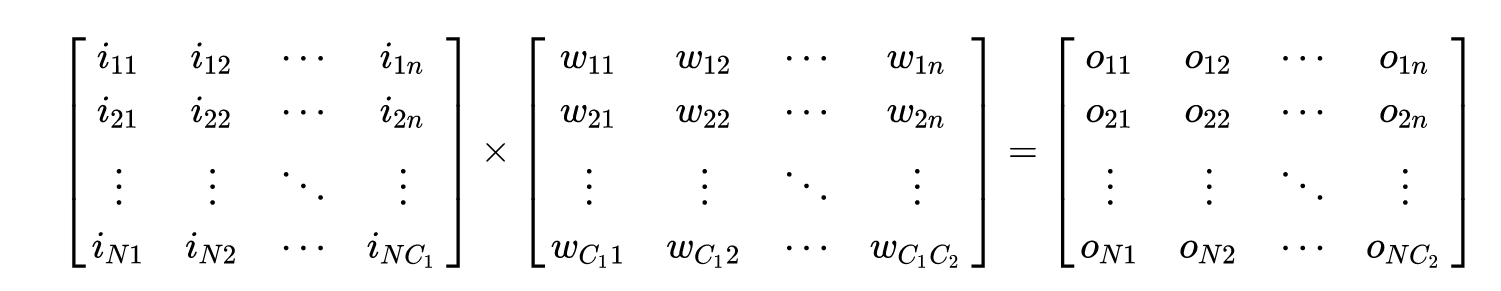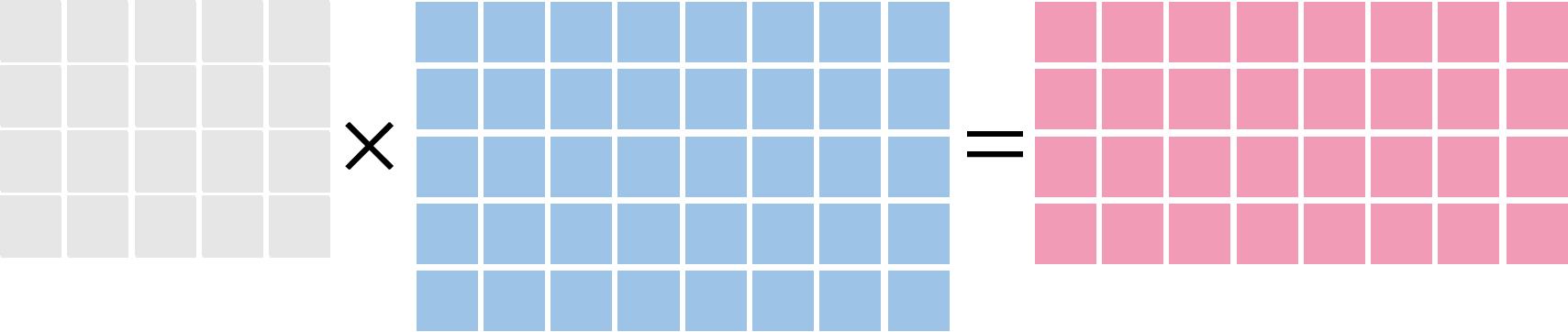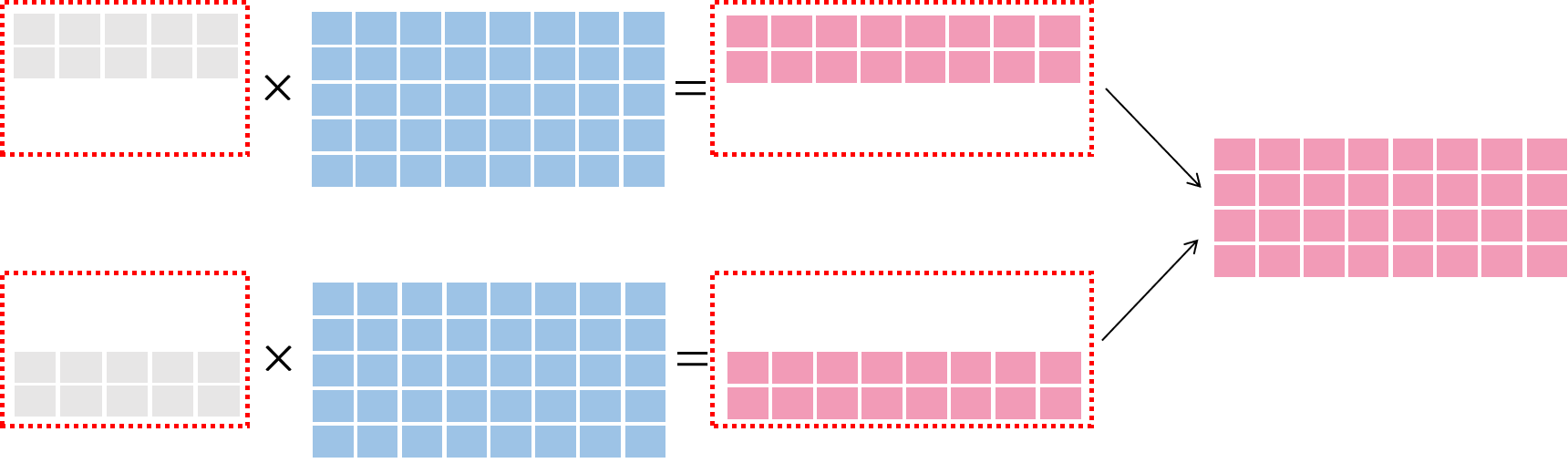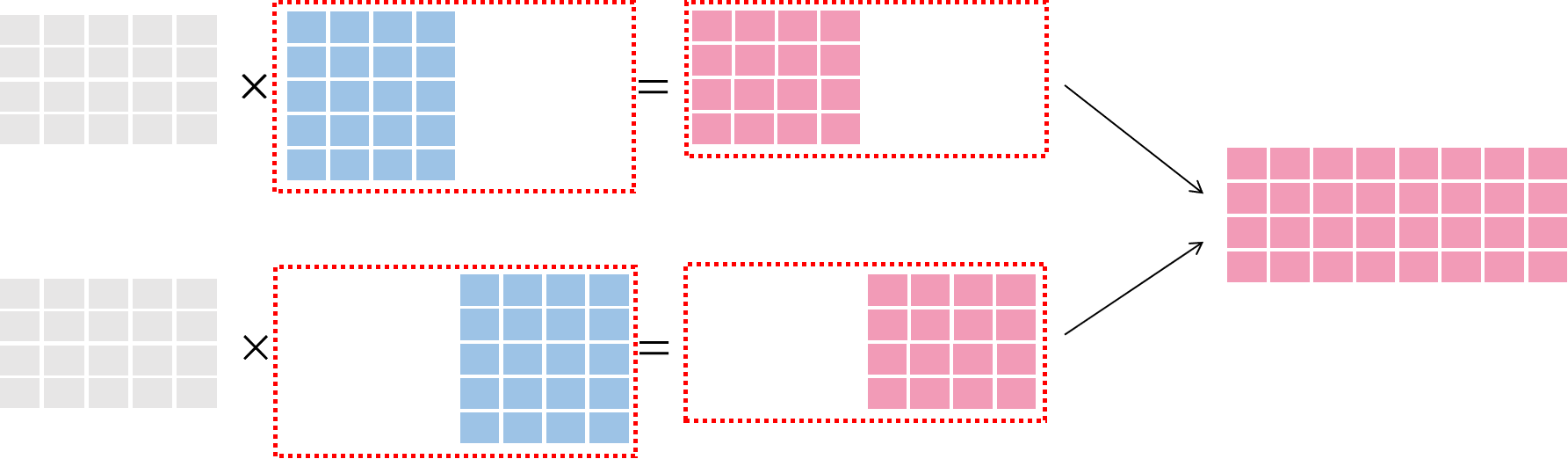# Consistent & Mirrored View

In distributed training, OneFlow provides two aspects for determining the relationship between data and model. There are `consistent` view and `mirrored` view.

• The difference and applicable scenario of data parallelism and model parallelism.

• The characteristics of `mirrored` view in distributed training.

• The characteristics of `consistent` view in distributed training.

## Data Parallelism and Model Parallelism.¶

In order to better understand `consistent` and `mirrored` in OneFlow, we need to understand the difference between data parallelism and model parallelism in distributed training.

In order to show the difference more visually, let's look at a simple Op: Matrix multiplication.

We assume that during training, there is an input matrix I and the output matrix O is obtained by multiplying matrix I with another matrix W.As shown in the figure, the size of I is (N, C1), the size of W is (C1, C2) and the size of O is (N, C2).

In machine learning, we can describe the matrixes as following:

• Matrix I is the input object, each row is a sample and each column represents the features of the sample.

• Matrix W represents the parameters of the model.

• Matrix O is the prediction result.

If `N` in the matrix I is very large, we have large-scale samples. If `C2` in matrix W is very large, it means we have a very complex model. If the scale and complexity reach a point, the single machine with a single device will not able to handle the training job. We might consider the distributed training. In a distributed training system, we can choose data parallelism and model parallelism.

In order to better understand data parallelism and model parallelism, we use the following figure as the demo of matrix multiplication:The first matrix in grey on the left-hand side of the equation is the input sample. Each row is a sample. The second matrix in blue on the left-hand side of the equation is the parameter(model).

In this section, we will see how the operators above are split in different ways in data parallelism and model parallelism.

### Data Parallelism Diagram¶

In data parallelism, the sample data are divided into small parts. The divided data will send to each training node and calculate with the complete models. Finally, we combine the information in each node. As shown in the figure below:### Model Parallelism Diagram¶

In model parallelism, the model will be divided. Complete data will be sent to each node and calculate with the divided model. Finally, we combine the model in each node. As shown in the figure below:In conclusion:

• In data parallelism, each node uses the same model to train and the data is divided.

• In model parallelism, each node receives the same data and the model is divided.

We will introduce two parallelism strategies in OneFlow (`mirrored` and `consistent`) and learn how to choose different parallelism methods in different strategies.

### Two Types of Placeholder¶

In use OneFlow build neural network and The Definition and Call of Job Function, we have already introduced the concept of `Placeholder` and `Blob`.

Actually, in the view of parallelism, the `Placeholder` of OneFlow can be divided into two types: Use `oneflow.typing.Numpy.Placeholder` and `oneflow.typing.ListNumpy.Placeholder` to construct the placeholder, which is corresponding to `Consistent` and `Mirrored`.

We will explain them in detail in the examples below.

## Using Mirrored View in OneFlow¶

Other frameworks like TensorFlow or Pytorch support `mirrored view`. The `mirrored view` of OneFlow is similar to them.

In mirrored view, the model are copied in each GPU, the graph building on each node is the same, thus we can only use data parallelism.

In OneFlow, the default strategy is consistent view, so you should use `default_logical_view` of `flow.function_config()` to define:

``````    func_config = flow.function_config()
func_config.default_logical_view(flow.scope.mirrored_view())
``````

In `mirrored_view`, we can only use data parallelism. When we call the job function, we need to divide the data evenly according to the amount of the devices and put the data after dividing it into a `list`. Every element in the `list` is the data to send to each device.

The return value type of job function is `oneflow.typing.ListNumpy`. Every element in the `list` is corresponding to the results of each device.

Concat all elements in the `list` can make a complete batch.

### Code¶

In the following code, we use `mirrored_view` with two devices to train.

Complete Code: mirrored_strategy.py

We will explain the key part of the code in detail in the following "code explanation" section.

### Code explanation¶

In the above code:

• Use `flow.config.gpu_device_num` to set device amount as 2.

``````flow.config.gpu_device_num(2)
``````

• `oneflow.typing.ListNumpy.Placeholder` defined the sample amount which is divided. And the relationship between `BATCH_SIZE_PER_GPU` and `BATCH_SIZE` is `BATCH_SIZE=BATCH_SIZE_PER_GPU×GPU_NUM`.

``````def train_job(
images: tp.ListNumpy.Placeholder((BATCH_SIZE_PER_GPU, 1, 28, 28), dtype=flow.float),
labels: tp.ListNumpy.Placeholder((BATCH_SIZE_PER_GPU,), dtype=flow.int32),
) -> tp.ListNumpy:
``````

• The data after dividing need to be stored in the `list` and passed to training functions. The number of elements in `list` need to be same as the amount of devices in the training process. The i-th element in `list` will be sent to the i-th device:

``````  images1 = images[:BATCH_SIZE_PER_GPU]
images2 = images[BATCH_SIZE_PER_GPU:]
labels1 = labels[:BATCH_SIZE_PER_GPU]
labels2 = labels[BATCH_SIZE_PER_GPU:]

imgs_list = [images1, images2]
labels_list = [labels1, labels2]

loss = train_job(imgs_list, labels_list)
``````

• The return result `loss` is a `list`, the number of elements in this `list` need to be same as the amount of devices in the training process. Then we concat them and print the `total_loss`.

`````` total_loss = np.array([*loss, *loss])
if i % 20 == 0:
print(total_loss.mean())
``````

## Use consistent view in OneFlow¶

We have already learned about the mirrored view, where samples will be distributed evenly while the models are the same in every device, and the results of each node need to be assembled to get the complete batch.

In addition to mirrored view, OneFlow also provides consistent view. Consistent view is one of the features of OneFlow. Compared with mirrored view, it has a great advantage.

OneFlow will use consistent view as default. We can declare it explicitly as the following code.

``````  config = flow.function_config()
config.default_distribute_strategy(flow.scope.consistent_view())
``````
The reason why consistent view is the main feature of OneFlow is that in OneFlow design, if we use `consistent_view`, multiple devices in a distributed system can get consistently in logic level from user's point of view. We use matrix multiplication as an example in the beginning of article, we only need focus on matrix multiplication itself on mathematics level. But in project, the issue of how to config and use model parallelism or data parallelism can be easily done in OneFlow. OneFlow will handle The data division in data parallelism, model division in model parallelism and serial logic issue quickly and efficiently.

In consistent view of OneFlow, we can choose model parallelism, data parallelism, or hybrid parallelism freely.

### Code Example¶

In the following code, we use consistent view and use two devices to train. The default parallelism method is data parallelism in consistent view. The issue of how to set model parallelism and hybrid parallelism in consistent view will be discussed in parallels features of OneFlow.

Complete code: consistent_strategy.py

### Code explanation¶

In above code:

• Use `flow.config.gpu_device_num` to set the amount of devices:

``````flow.config.gpu_device_num(2)
``````

• Use `tp.Numpy.Placeholder` to define the placeholder in consistent view. Because the blob of `Numpy.Placeholder` represent the op and placeholder in logic. Thus. the BATCH_SIZE is the sum of samples, without artificial split or combination

``````@flow.global_function(type="train")
def train_job(
images: tp.Numpy.Placeholder((BATCH_SIZE, 1, 28, 28), dtype=flow.float),
labels: tp.Numpy.Placeholder((BATCH_SIZE,), dtype=flow.int32),
) -> tp.Numpy:
``````

• The job function is called directly to obtain the training results. The splitting and concatenating in the distributed training are completed automatically by OneFlow. In the consistent view, there are few differences between the single-machine training program and the distributed training program.

``````for i, (images, labels) in enumerate(zip(train_images, train_labels)):
loss = train_job(images, labels)
if i % 20 == 0:
print(loss.mean())
``````

## More extension¶

With the development of machine learning theory and practice, there are many models unable to train in a single device or by data parallelism only.

Adopting `consistent` view in OneFlow, the above problems can be solved well through free selection and combination of parallel methods. We will introduce in parallel features of OneFlow.